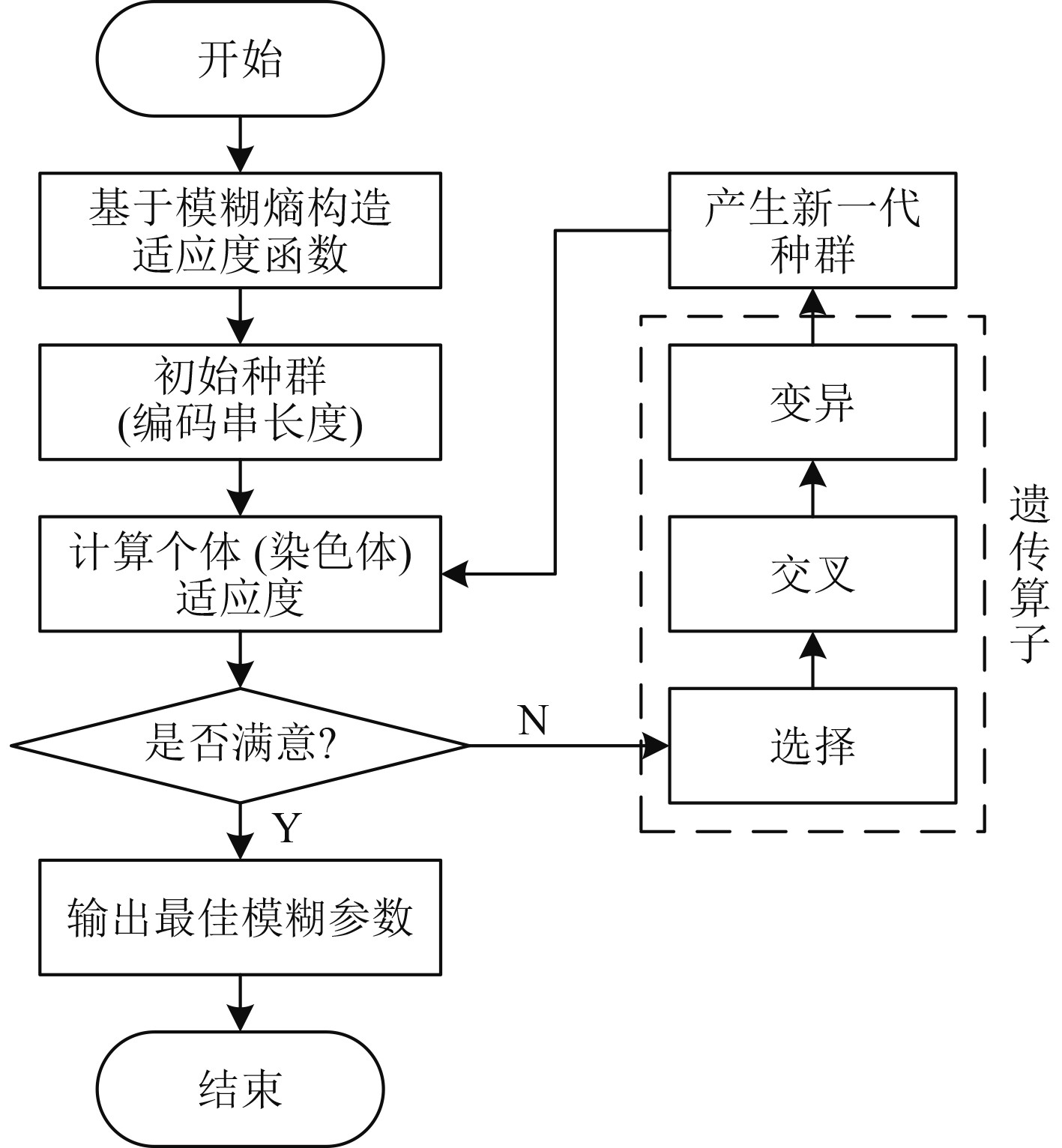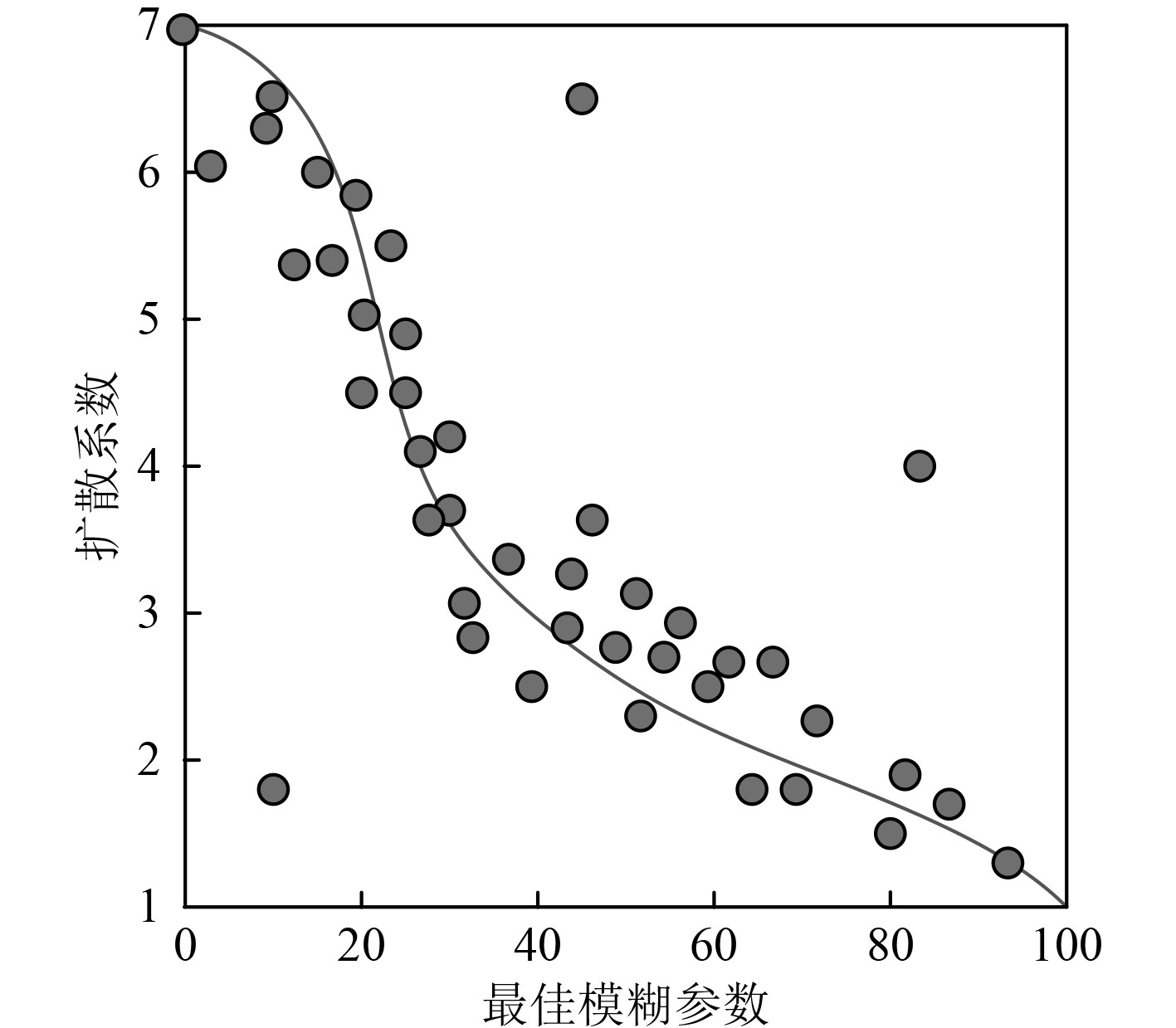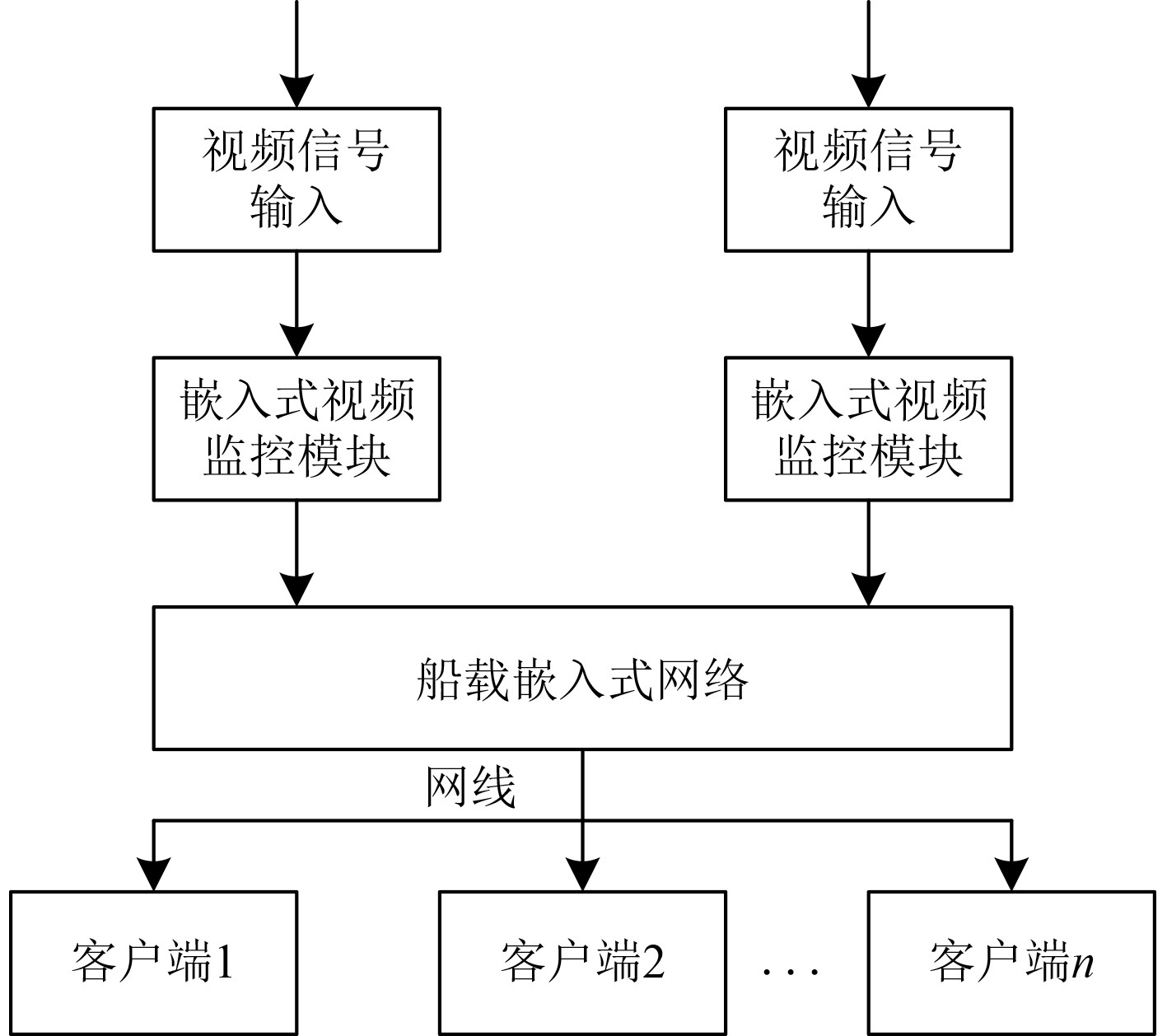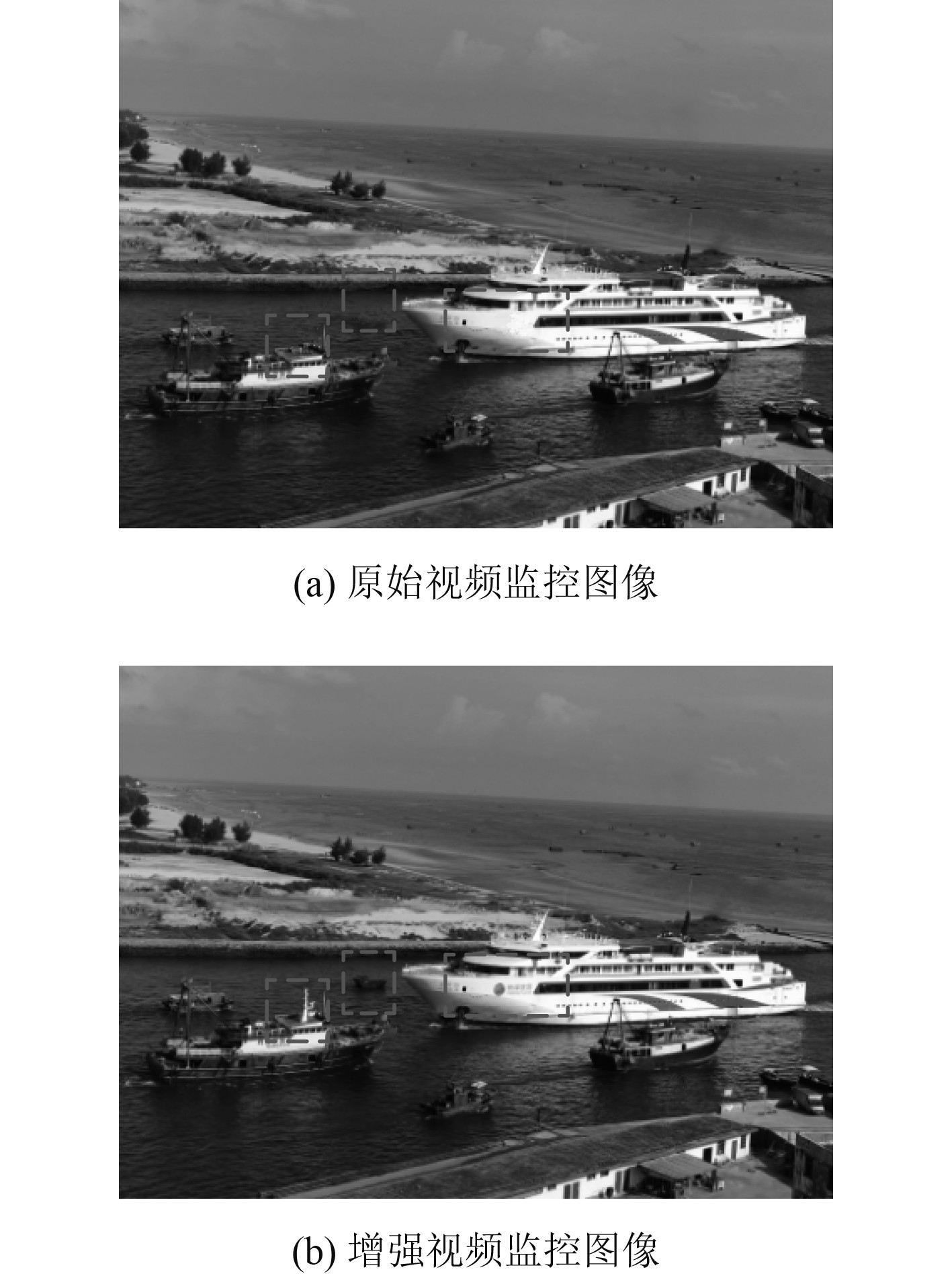﻿ 船载嵌入式网络视频监控图像模糊细节增强系统
 舰船科学技术2022, Vol. 44Issue (19): 162-165    DOI: 10.3404/j.issn.1672-7649.2022.19.033PDF

1. 太原工业学院 电子工程系，山西 太原 030008;
2. 中北大学信息探测与处理山西省重点实验室，山西 太原 030051

Ship borne embedded network video surveillance image fuzzy detail enhancement system
ZHANG Jun-sheng1,2, HE Ying-feng1, YANG Peng1, TONG Xiao-gang1
1. Department of Electronic engineering, Taiyuan Institute of Technology, Taiyuan 030008, China;
2. Shanxi Key Laboratory of Signal Capturing and Processing, North University of China, Taiyuan 030051, China
Abstract: The widespread application of onboard embedded network provides a strong support for the video monitoring of ships and their navigation environment, and provides a large amount of image data for the decision-making of ships' navigation. Due to the influence of weather environment, equipment vibration and other factors, image details are missing, which restricts the application of video surveillance images. The video surveillance image is preprocessed through the dynamic range stretching mode. On this basis, the fuzzy set theory is used to calculate the detail fuzzy entropy of the video surveillance image. The genetic algorithm is used to determine the best fuzzy parameters, calculate the diffusion coefficient, combine it with the fuzzy gradient field of the video surveillance image, apply the variational method to solve the modified membership function, and convert the video surveillance image from the fuzzy domain to the gray domain through inverse transformation, The shipboard embedded network video surveillance image with enhanced details can be obtained. The experimental data shows that the maximum information entropy of the enhanced video surveillance image obtained by the application of the design system is 9.45, which fully proves that the design system has better details enhancement effect.
Key words: embedded network     image enhancement     blur details     ship video monitoring     complex background     atmospheric scattering model
0 引　言

1 视频监控图像模糊细节增强系统 1.1 视频监控图像预处理

 $\left[ {\begin{array}{*{20}{c}} H&S&I \end{array}} \right] = \xi _{RGB}^{HSI}\left[ {\begin{array}{*{20}{c}} {R'}&{G'}&{B'} \end{array}} \right]。$ (1)

1.2 视频监控图像细节模糊熵计算

 $E\left( {{f'_i}} \right) = 1 - \frac{{{\chi _e}{\delta _\alpha }\left( {{f'_i},{f'_j}} \right)}}{{{n^{1/\alpha }}}}。$ (2)

1.3 最佳模糊参数确定图 1 最佳模糊参数确定程序图 Fig. 1 Program diagram for determining the optimal fuzzy parameters

 $K\left({x}_{i}\right)=\frac{E\left({f}^{\prime }_{i}\right)}{M}+{\varepsilon }_{i}=\frac{1}{M}-\frac{{\chi }_{e}{\delta }_{\alpha }\left({f}^{\prime }_{i},{f}^{\prime }_{j}\right)}{M\times {n}^{1/\alpha }}+{\varepsilon }_{i}。$ (3)

 ${x'_i} = \frac{{{x_i} \times {P_1} \times {P_2}}}{{{\tau ^o}}}。$ (4)

1.4 视频监控图像模糊细节增强

 $\eta \left( {{{\hat \chi }_e}} \right) = 1 + \frac{\gamma }{{1 + {{\left( {{{\hat \chi }_e}/\kappa } \right)}^2}}}。$ (5)图 2 最佳模糊参数与扩散系数关系拟合曲线图 Fig. 2 Fitting curve of the relationship between the best fuzzy parameter and diffusion coefficient

 $\beta \left( {x,y} \right) = \frac{{R\left( {x,y} \right) - {R_{\min }}\left( {x,y} \right)}}{{{R_{\max }}\left( {x,y} \right) - {R_{\min }}\left( {x,y} \right)}}。$ (6)

 $T\left( {x,y} \right) = \eta \left( {{{\hat \chi }_e}} \right) * \varGamma \left( {x,y} \right)。$ (7)

 $\Upsilon \left[ {\beta '\left( {x,y} \right)} \right] = \int {\int {{{\left| {\nabla \beta \left( {x,y} \right) - T\left( {x,y} \right)} \right|}^2}{\rm{d}}x{\rm{d}}y} }。$ (8)

2 仿真实验 2.1 实验准备图 3 船载嵌入式网络结构示意图 Fig. 3 Schematic diagram of shipborne embedded network structure

 $G\left( {i,j} \right) = {\rm{Med}}\left\{ {F\left( {i + s,j + t} \right),\left( {s,t} \right) \in M\left( {i,j} \right)} \right\}。$ (9)

 $D\left( {i,j} \right) = G\left( {i,j} \right) - {\mu ^o}{\nabla ^2}G\left( {i,j} \right)。$ (10)

2.2 实验结果分析图 4 视频监控图像模糊细节增强结果示意图 Fig. 4 Schematic diagram of fuzzy detail enhancement results of video surveillance image

3 结　语

  王兴瑞, 朴燕, 王雨墨. 复合残差网络在低照度图像增强中的技术研究[J]. 液晶与显示, 2022, 37(5): 508-518.  刘海艳, 李俊敏. 集成射频与视频监控的交通流参数检测技术[J]. 电子技术应用, 2021, 47(4): 77–81.  丁伟祥. 基于Web身份特征的网络视频监控设备识别[J]. 沈阳工业大学学报, 2020, 42(4): 427–431.  王卓, 张长胜, 钱俊兵. 边缘细分的动态参数模糊C-均值图像分割算法[J]. 南京理工大学学报, 2020, 44(3): 288–295.  王小芳, 邹倩颖, 彭林子, 等. 融合模糊聚类的蚁群图像增强算法[J]. 数据采集与处理, 2020, 35(3): 506–515.  朱颖, 王昕, 王爱平, 等. 基于MVOtsu和对数型模糊隶属度函数的电力设备NSST域红外图像增强[J]. 高压电器, 2020, 56(9): 179–185.  曹令, 刘桂华, 邓豪, 等. 基于局部关联信息的视频监控图像中γ辐射噪斑的消除方法[J]. 原子能科学技术, 2022, 56(7): 1413–1422.  曹锦纲, 李金华, 郑顾平. 基于生成式对抗网络的道路交通模糊图像增强[J]. 智能系统学报, 2020, 15(3): 491–498.